• 时间复杂度公示： 下面分析一段代码： 2、3、4行每一行都需要1个单位的执行时间，5、6行循环了N边，需要2N个单位时间，7、8行执行了n^2遍，所以一共需要执行时间：（2N ^2 + 2N + 3 ）*单位时间。 我们用T(n) 表示...
时间复杂度公示：下面分析一段代码：2、3、4行每一行都需要1个单位的执行时间，5、6行循环了N边，需要2N个单位时间，7、8行执行了n^2遍，所以一共需要执行时间：（2N ^2 + 2N + 3 ）*单位时间。 我们用T(n) 表示，O表示T(n)与f(n)成正比（单位时间），所以表达式可以写成：T(n) = O(2N ^2 + 2N + 3 ) ,这就是大O时间复杂度表示法，由于N无穷大，所以只需要表示最大量级即可，即：T(n) = O(N ^2 )
时间复杂度分析：
1、只关注循环执行次数最多的一段代码； 2、加法法则：总复杂度等于量级最大的那段代码的复杂度； 例如：时间复杂度：T(n) = O(N+N ^2 ) = O（N ^2 ）3、乘法法则：嵌套代码的复杂度等于嵌套内外代码的复杂度乘积； 例如： 以下算法时间复杂度为：T(n) = O(N X N)=O(N ^2 )展开全文• 时间复杂度和空间复杂度 时间复杂度：所有语句执行的次数和T(n)。 一般不同的输入会有不同的时间复杂度。 计算方法：先找到循环变量i 例如 for(i=1;i<n;i=i*2) std::cout<<i<<std::endl...
算法评价：

时间复杂度和空间复杂度

时间复杂度：所有语句执行的次数。 用T(n)来衡量时间复杂度。 一般不同的输入会有不同的时间复杂度。

计算方法：先找到循环变量i

例1
for(i=1;i<n;i=i*2)
std::cout<<i<<std::endl;

确定循环终止条件：

i

<

=

n

i<=n

循环变量的增加方式：

i

=

i

∗

2

i=i*2

假设执行了t次，

i

=

2

t

i=2^{t}

，代入1

2

t

<

=

n

2^{t}<=n

==>

t

<

=

log

⁡

2

n

t<=\log_2n

T(n)=

log

⁡

2

n

\log_2n

=

O

(

log

⁡

2

n

)

O(\log_2n)

例2.一算法所需时间由下述递归方程表示，求该算法时间复杂度。

T

(

n

)

=

{

1

,

n

=

1

2

T

(

n

/

2

)

+

n

,

n

>

1

T(n)=\begin{cases} 1& ,n=1\\ 2T(n/2)+n& ,n>1\\ \end{cases}

n是问题规模，为简单起见n为2的整数次幂。 解：

令

n

=

2

k

,

则

k

=

0

时

，

T

(

2

k

)

=

1

;

令n=2^k,则k=0时，T(2^k)=1;

k

>

0

时

，

T

(

2

k

)

=

2

T

(

2

k

−

1

)

+

2

k

,

k>0时，T(2^k)=2T(2^{k-1})+2^k,

T

(

2

k

)

2

k

=

T

(

2

k

−

1

)

2

k

−

1

+

1

,

\frac{T(2^k)}{2^k}=\frac{T(2^{k-1})}{2^{k-1}}+1,

令

y

k

=

T

(

2

k

)

2

k

=

>

y

k

=

y

k

−

1

+

1

,

又

y

0

=

1

,

令y_k=\frac{T(2^k)}{2^k}=>y_k=y_{k-1}+1,又y_0=1,

y

k

=

k

+

1

,

得

T

(

2

k

)

=

2

k

(

k

+

1

)

y_k=k+1,得T(2^k)=2^k(k+1)

T

(

n

)

=

n

(

log

⁡

2

n

+

1

)

=

n

log

⁡

2

n

+

n

T(n)=n(\log_2n+1)=n\log_2n+n

得时间复杂度级别为

O

(

n

log

⁡

2

n

)

O(n\log_2n)


展开全文• 时间复杂度能稳定在O(NlogN)，时间复杂度证明如下图所示。不想快速排序，如果碰到有序的数组，快速排序会退化到O(N^2) 归并排序空间复杂度O(NlogN)，证明如下 public class Solution { public static Integer[]...
归并排序有个merge()方法，是将两个排好序的数组合并成一个有序数组。时间复杂度能稳定在O(NlogN)，时间复杂度证明如下图所示。不想快速排序，如果碰到有序的数组，快速排序会退化到O(N^2)  归并排序空间复杂度O(NlogN)，证明如下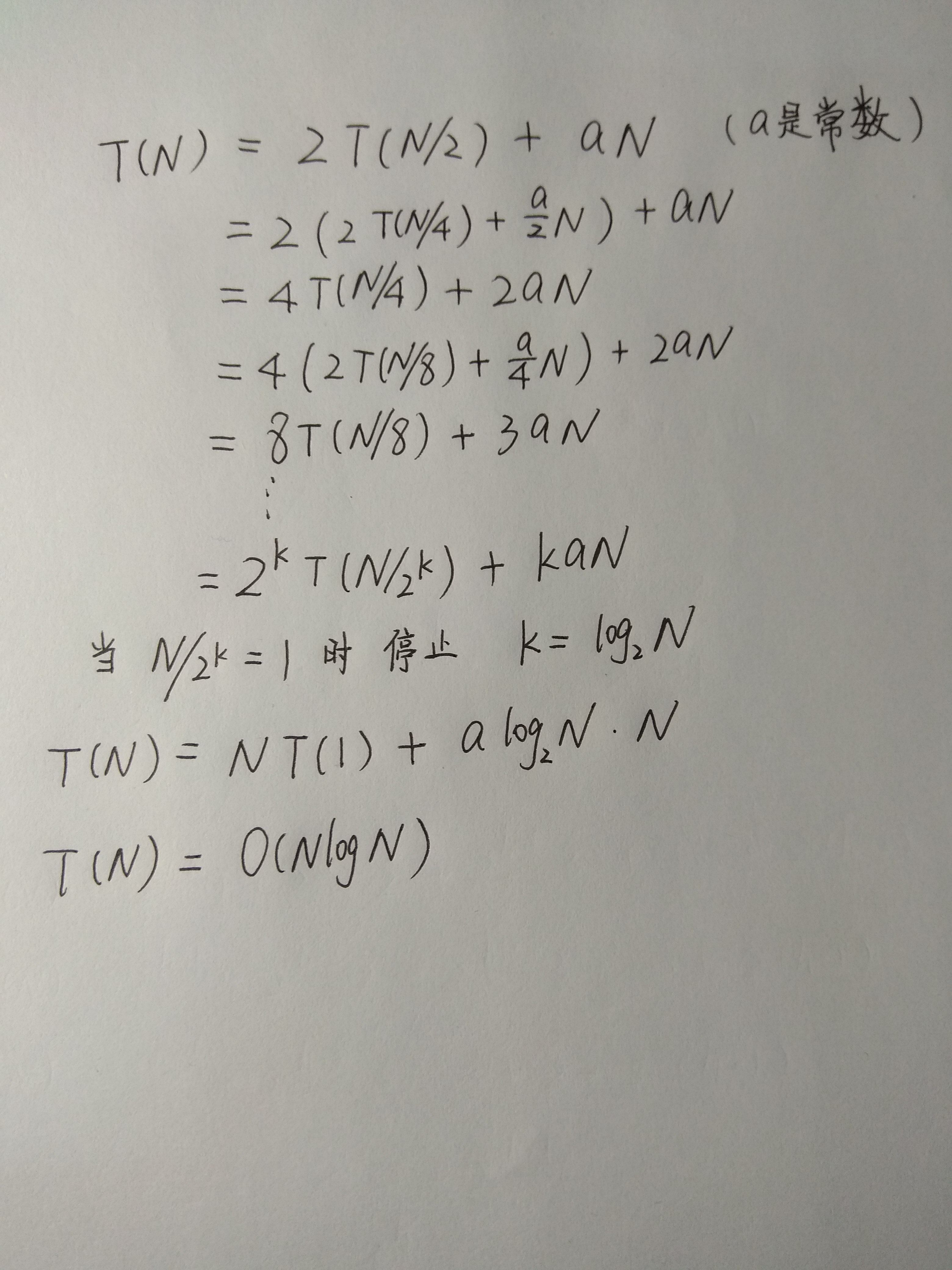public class Solution {
public static Integer[] tmp;

public static void main(String[] args) {
Integer[] a = new Integer[]{3,4,2,6,5,7,9,1,8,7};
tmp = new Integer[a.length];
Mergesort(a, 0, 9, tmp);
System.out.println(Arrays.toString(a));
return;
}

public static void Mergesort(Integer[] a,int start, int end, Integer[] tmp){
if (end == start){
return;
}
int mid = (end-start)/2 + start;
Mergesort(a, start, mid, tmp);
Mergesort(a, mid+1, end, tmp);
Merge(a, start, mid, end, tmp);
}
public static void Merge(Object[] a, int start, int mid, int end, Object[] tmp){
int index = 0;
int i = start, j = mid+1;
while (i <= mid && j<= end){
if (((Comparable)a[i]).compareTo(a[j]) <= 0){
tmp[index++] = a[i];
++i;
}
else {
tmp[index++] = a[j];
++j;
}
}
while (i <= mid){
tmp[index++] = a[i];
i++;
}
while (j <= end){
tmp[index++] = a[j];
++j;
}
for (int k = 0; k< index;++k){
a[start+k] = tmp[k];
}
}
}
展开全文• 样本熵计算时间序列的复杂度，熵值高，则复杂度高，熵值低，复杂度低。
• ## 一套图 搞懂“时间复杂度”

万次阅读 多人点赞 2019-06-04 13:12:15
是我到目前为止所看到的关于时间复杂度介绍的最好的文章，简介 清晰 明了。 所以拿来po出来 仅供学习交流，如侵则删。 现已将此文收录至：《数据结构》C语言版 (清华严蔚敏考研版） 全书知识梳理 正文： ...
写在前面：
这篇文章是在公众号： 程序员小灰 中发布的。是我到目前为止所看到的关于时间复杂度介绍的最好的文章，清晰明了。
所以拿来po出来 仅供学习交流，如侵则删。
现已将此文收录至： 《数据结构》C语言版 (清华严蔚敏考研版） 全书知识梳理

同类好文： 8种方法优雅地利用C++编程从1乘到20
从B站 (哔哩哔哩) 泄露的源码里发现了B站视频推荐的秘密

正文：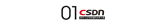时间复杂度的意义

究竟什么是时间复杂度呢？让我们来想象一个场景：某一天，小灰和大黄同时加入了一个公司......一天过后，小灰和大黄各自交付了代码，两端代码实现的功能都差不多。大黄的代码运行一次要花100毫秒，内存占用5MB。小灰的代码运行一次要花100秒，内存占用500MB。于是......由此可见，衡量代码的好坏，包括两个非常重要的指标：
1.运行时间；
2.占用空间。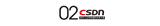基本操作执行次数

关于代码的基本操作执行次数，我们用四个生活中的场景，来做一下比喻：
场景1：给小灰一条长10寸的面包，小灰每3天吃掉1寸，那么吃掉整个面包需要几天？答案自然是 3 X 10 = 30天。
如果面包的长度是 N 寸呢？
此时吃掉整个面包，需要 3 X n = 3n 天。
如果用一个函数来表达这个相对时间，可以记作 T（n） = 3n。
场景2：给小灰一条长16寸的面包，小灰每5天吃掉面包剩余长度的一半，第一次吃掉8寸，第二次吃掉4寸，第三次吃掉2寸......那么小灰把面包吃得只剩下1寸，需要多少天呢？
这个问题翻译一下，就是数字16不断地除以2，除几次以后的结果等于1？这里要涉及到数学当中的对数，以2位底，16的对数，可以简写为log16。
因此，把面包吃得只剩下1寸，需要 5 X log16 = 5 X 4 = 20 天。
如果面包的长度是 N 寸呢？
需要 5 X logn = 5logn天，记作 T（n） = 5logn。
场景3：给小灰一条长10寸的面包和一个鸡腿，小灰每2天吃掉一个鸡腿。那么小灰吃掉整个鸡腿需要多少天呢？答案自然是2天。因为只说是吃掉鸡腿，和10寸的面包没有关系 。
如果面包的长度是 N 寸呢？
无论面包有多长，吃掉鸡腿的时间仍然是2天，记作 T（n） = 2。
场景4：给小灰一条长10寸的面包，小灰吃掉第一个一寸需要1天时间，吃掉第二个一寸需要2天时间，吃掉第三个一寸需要3天时间.....每多吃一寸，所花的时间也多一天。那么小灰吃掉整个面包需要多少天呢？
答案是从1累加到10的总和，也就是55天。
如果面包的长度是 N 寸呢？
此时吃掉整个面包，需要 1+2+3+......+ n-1 + n = (1+n)*n/2 = 0.5n^2 + 0.5n。
记作 T（n） = 0.5n^2 + 0.5n。上面所讲的是吃东西所花费的相对时间，这一思想同样适用于对程序基本操作执行次数的统计。刚才的四个场景，分别对应了程序中最常见的四种执行方式：
场景1：T（n） = 3n，执行次数是线性的。
void eat1(int n){
for(int i=0; i<n; i++){;
System.out.println("等待一天");
System.out.println("等待一天");
System.out.println("吃一寸面包");
}
}
vo

场景2：T（n） = 5logn，执行次数是对数的。
void eat2(int n){
for(int i=1; i<n; i*=2){
System.out.println("等待一天");
System.out.println("等待一天");
System.out.println("等待一天");
System.out.println("等待一天");
System.out.println("吃一半面包");
}
}

场景3：T（n） = 2，执行次数是常量的。
void eat3(int n){
System.out.println("等待一天");
System.out.println("吃一个鸡腿");
}

场景4：T（n） = 0.5n^2 + 0.5n，执行次数是一个多项式。
void eat4(int n){
for(int i=0; i<n; i++){
for(int j=0; j<i; j++){
System.out.println("等待一天");
}
System.out.println("吃一寸面包");
}
}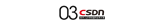渐进时间复杂度

有了基本操作执行次数的函数 T（n），是否就可以分析和比较一段代码的运行时间了呢？还是有一定的困难。
比如算法A的相对时间是T（n）= 100n，算法B的相对时间是T（n）= 5n^2，这两个到底谁的运行时间更长一些？这就要看n的取值了。
所以，这时候有了渐进时间复杂度（asymptotic time complexity）的概念，官方的定义如下：
若存在函数 f（n），使得当n趋近于无穷大时，T（n）/ f（n）的极限值为不等于零的常数，则称 f（n）是T（n）的同数量级函数。
记作 T（n）= O（f（n）），称O（f（n）） 为算法的渐进时间复杂度，简称时间复杂度。
渐进时间复杂度用大写O来表示，所以也被称为大O表示法。如何推导出时间复杂度呢？有如下几个原则：
如果运行时间是常数量级，用常数1表示；  只保留时间函数中的最高阶项；  如果最高阶项存在，则省去最高阶项前面的系数。
让我们回头看看刚才的四个场景。
场景1：
T（n） = 3n
最高阶项为3n，省去系数3，转化的时间复杂度为：
T（n） =  O（n）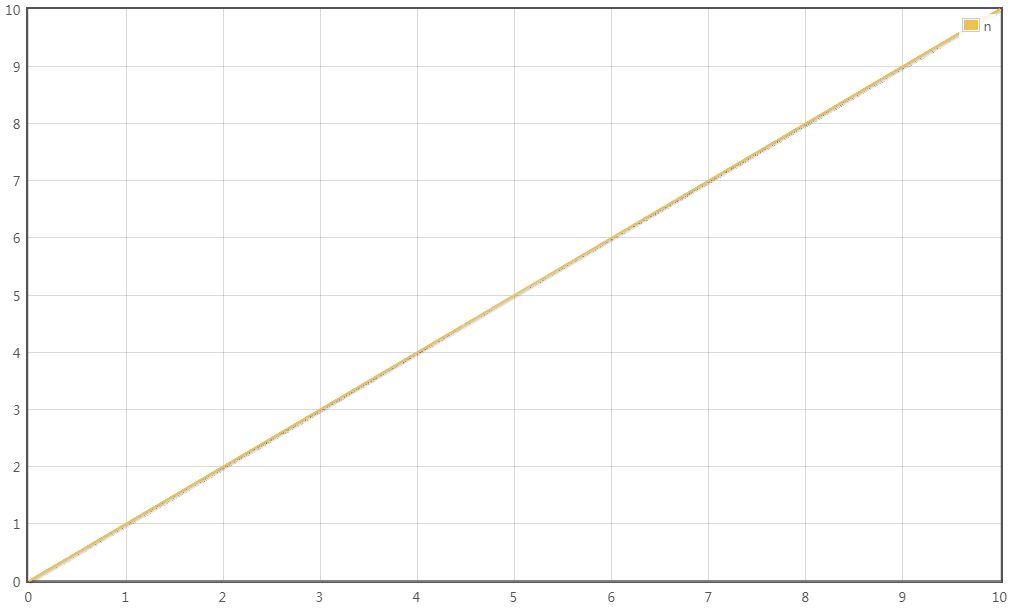场景2：
T（n） = 5logn
最高阶项为5logn，省去系数5，转化的时间复杂度为：
T（n） =  O（logn）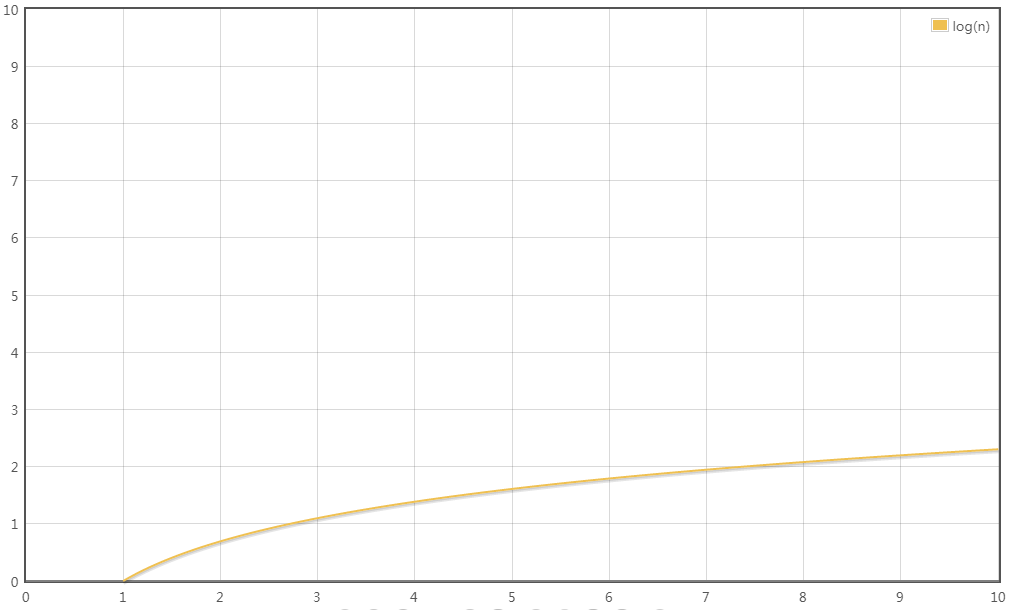场景3：
T（n） = 2
只有常数量级，转化的时间复杂度为：
T（n） =  O（1）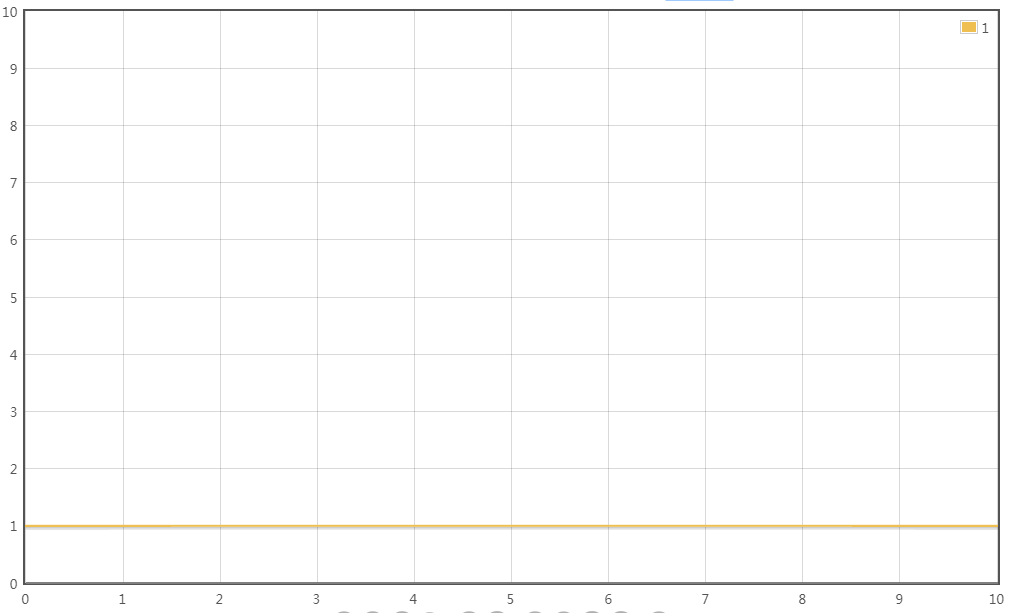场景4：
T（n） = 0.5n^2 + 0.5n
最高阶项为0.5n^2，省去系数0.5，转化的时间复杂度为：
T（n） =  O（n^2）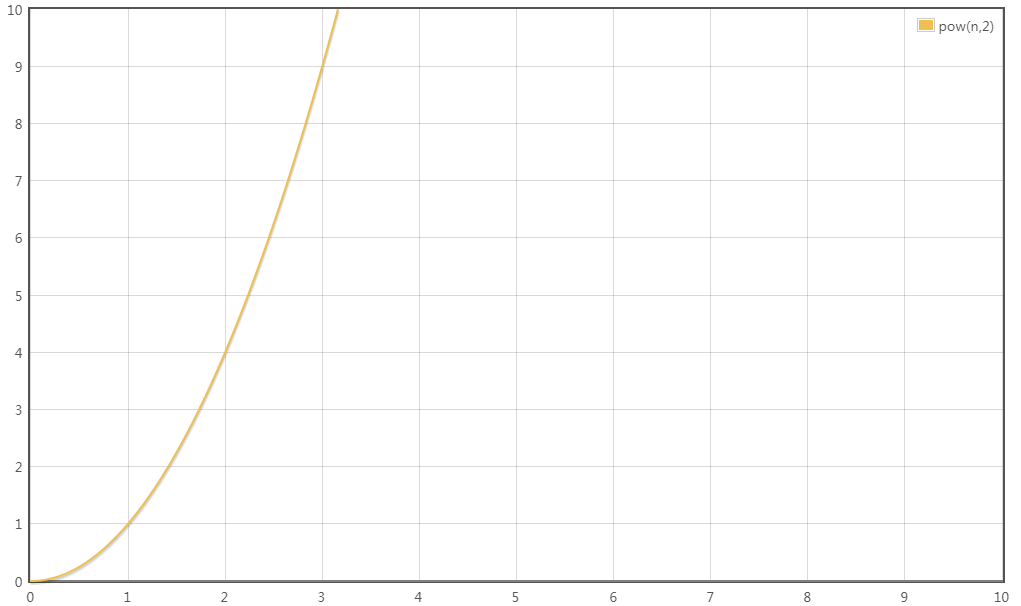这四种时间复杂度究竟谁用时更长，谁节省时间呢？稍微思考一下就可以得出结论：
O（1）< O（logn）< O（n）< O（n^2）
在编程的世界中有着各种各样的算法，除了上述的四个场景，还有许多不同形式的时间复杂度，比如：
O（nlogn）, O（n^3）, O（m*n），O（2^n），O（n！）
今后遨游在代码的海洋里，我们会陆续遇到上述时间复杂度的算法。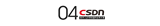时间复杂度的巨大差异我们来举过一个栗子：
算法A的相对时间规模是T（n）= 100n，时间复杂度是O(n)
算法B的相对时间规模是T（n）= 5n^2，时间复杂度是O(n^2)
算法A运行在小灰家里的老旧电脑上，算法B运行在某台超级计算机上，运行速度是老旧电脑的100倍。
那么，随着输入规模 n 的增长，两种算法谁运行更快呢？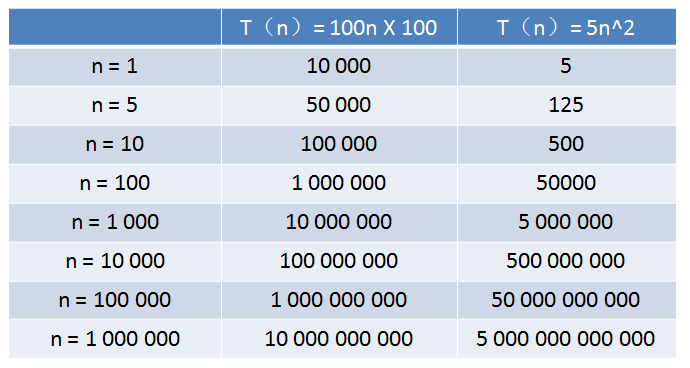从表格中可以看出，当n的值很小的时候，算法A的运行用时要远大于算法B；当n的值达到1000左右，算法A和算法B的运行时间已经接近；当n的值越来越大，达到十万、百万时，算法A的优势开始显现，算法B则越来越慢，差距越来越明显。
这就是不同时间复杂度带来的差距。如果感觉还不错，点个赞↗ 支持一下吧 ~
随后还会不定期更新同类型文章，欢迎订阅关注我的博客 ~
下一篇：400+条实用C/C++框架、库、工具整理 ，你能想到的都在这里了
上一篇： Facebook前身 哈佛大学"选美"网站核心算法 -- ELO等级分制度(附源码)
展开全文算法
• 时间复杂度是学习算法的基石，今天我们来聊聊为什么要引入时间复杂度，什么是时间复杂度以及如何去一个算法的时间复杂度 一、刻画算法的运行时间 某日，慧能叫来了一尘打算给他补习补习一下基础知识，只见克写了...算法
• 算法分析神器—时间复杂度 一套图 搞懂“时间复杂度” 目录 一、代码消耗的的时间单位分析 二、什么是时间复杂度？ 三、计算时间复杂度？ 一、得出运行时间的函数  二、对函数进行简化  四、时间复杂度排行 五、...
• 时间复杂度 foriinrange(n): print('Hello world') # O(n) for i in range(n): for j in range(n): # O(n^2) i=1 while(i<N): i=i*2 # O(logN) forjinrange(n): i=1 while(i<N): i=i*2 # O(nlogN) ...
• ## 代码圈复杂度

千次阅读 2020-02-24 19:24:22
复杂度 (Cyclomatic complexity) 是一种代码复杂度的衡量标准，也称为条件复杂度或循环复杂度，它可以用来衡量一个模块判定结构的复杂程度，数量上表现为独立现行路径条数，也可理解为覆盖所有的可能情况最少使用...
• 文章目录数据结构与算法概述复杂度分析大O复杂度表示法时间复杂度分析几种常见时间复杂度实例分析空间复杂度分析复杂度坐标图复杂度分析的四个知识点 数据结构与算法概述 什么是数据结构？什么是算法？ 从广义上讲...算法
• 时间复杂度 时间频度 一个算法执行所耗费的时间，从理论上是不能出来的，必须上机运行测试才能知道。但我们不可能也没有必要对每个算法都上机测试，只需知道哪个算法花费的时间多，哪个算法花费的时间少就可以了。...
• 一、什么是时间复杂度 二、时间复杂度的计算 单个循环体的推导法则 多重循环体的推导法则 多个时间复杂度的推导法则 条件语句的推导法则 习题练习 一、基础题 二、进阶题 三、再次进阶 一、什么是时间...
• 代码执行时间随数据规模增长的变化趋势, 也叫作渐进时间复杂度，简称时间复杂度。T(n) = O(f(n)) (1) 我们在分析一个算法、一段代码时间复杂度的时候，也只关注循环执行次数最多的那一段代码就可以了。这段核心...
• 一、什么是时间复杂度？ 一个语句的频度是指该语句在算法中重复执行的次数，算法中所有语句的频度之和是关于问题规模n的函数T(n)，时间复杂度就是分析T(n)的数量级来得到的。算法的执行时间与T(n)的数量级成正比，...
• Java中代码时间复杂度 四个场景，分别对应了程序中最常见的四种执行方式： 场景1 T（n） = 3n，执行次数是线性的 场景2： T（n） = 5logn，执行次数是对数的 场景3： T（n） = 2，执行次数是常量的 场景4： T...JAVA
• //这句代码影响时间复杂度（每次循环加一个累加的变量） } 时间复杂度为O(√n)。 i=1,k=100; while(i<=n)//这个n对时间复杂度影响不大，看成任一常数即可 { k+=1; i+=2;//这句代码影响时间复杂度（每次循环...数据结构 算法
• ## 时间复杂度怎么算？

千次阅读 多人点赞 2020-12-31 13:08:24
时间复杂度计算时间复杂度计算案例大O表示法 时间复杂度：估算程序指令执行的次数 时间复杂度计算案例 注意：为了方便，省略了方法的修饰，只保留了方法名 method1(){ System.out.println("祝你看了这篇文章"); //...java 数据结构 算法 c语言 c++
• 下面代码段的时间复杂度是（）。 i=1; while( i<=n ) i=i*3; A. O(n) B. O(n^2) C. O(1) D. O(log3n) 正确答案：D 解析： 假设循环次数是x i = 1, 3, 9, 27, 81 ，i = 3^x 条件是i <= n 即3^...PAT
• 算法的时间复杂度和空间复杂度 1、时间复杂度 （1）时间频度 一个算法执行所耗费的时间，从理论上是不能出来的，必须上机运行测试才能知道。但我们不可能也没有必要对每个算法都上机测试，只需知道哪个算法花费的...算法 空间复杂度...